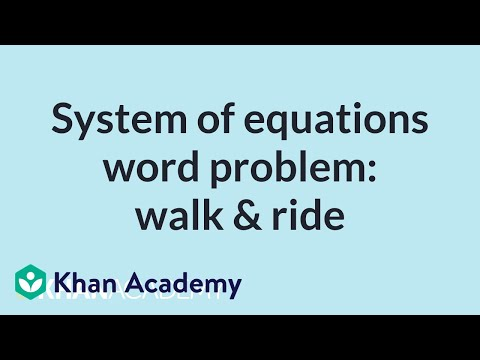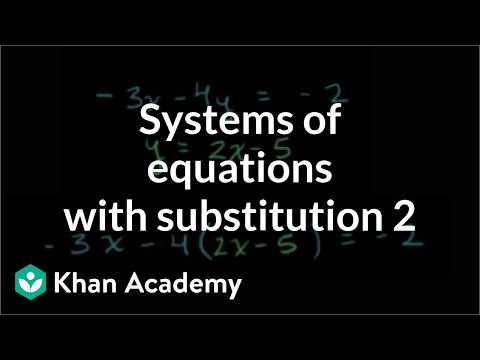# Qtr 2, Lesson 3: Writing Systems of Linear Equations

##By Stan Lisle

How to solve problems using two equations and two variables.# Systems of linear equations word problems

##By Khan Academy

Sal answers a word problem about a toy factory and finds that it has no solution.# Systems of linear equations word problems

##By Khan Academy

Sal solves a word problem about distances walking and riding bus to school, by creating a system of equations and solving it.# Row Reducing a Matrix - Systems of Linear Equations - Part 1

##By PatrickJMT

Visit : http://bit.ly/pTquLB for your 14 day free trial at Thinkwell! Videos, Quizzes, Tests and More! Row Reducing a Matrix - Systems of Linear Equations - Part 1. Basic notation and procedure as well as a full example are shown. The last part of the second part got cut off, but is finished in another video!!! For more free math videos, visit http://PatrickJMT.com Austin Math Tutor, Austin Math Tutoring, Austin Algebra Tutor, Austin Calculus Tutor# Systems of linear equations word problems

##By Khan Academy

Sal answers a word problem about a vegetable farmer and finds that it has infinite solutions.# Solve systems of linear equations with elimination (basic)

##By Khan Academy

Sal solves the following system of equations by eliminating x: x-4y=-18 and -x+3y=11.# Row Reducing a Matrix - Systems of Linear Equations - Part 1

##By PatrickJMT

Visit : http://bit.ly/pTquLB for your 14 day free trial at Thinkwell! Videos, Quizzes, Tests and More! Row Reducing a Matrix - Systems of Linear Equations - Part 1. Basic notation and procedure as well as a full example are shown. The last part of the second part got cut off, but is finished in another video!!! For more free math videos, visit http://PatrickJMT.com Austin Math Tutor, Austin Math Tutoring, Austin Algebra Tutor, Austin Calculus Tutor# Systems of linear equations word problems

##By Khan Academy

Sal answers a word problem about a toy factory and finds that it has no solution.# Algebra I Help: Solving Systems of Linear Equations Part II Graphing 2/3

##By yourteachermathhelp

The instructor uses a white board for demonstration and this video is suitable for high school students. Students learn to solve a system of linear equations by graphing. The first step is to graph each of the given equations then find the point of intersection of the two lines which is the solution to the system of equations. If the two lines are parallel then the solution to the system is the null set. If the two given equations represent the same line then the solution to the system is the equation of that line.# Systems of Linear Equations - Inconsistent Systems Using Elimination by Addition - Example 1

##By PatrickJMT

YouTube presents Systems of Linear Equations - Inconsistent Systems Using Elimination by Addition - Example 1 an educational video resources on math.# Solve systems of linear equations with substitution

##By Khan Academy

Sal solves the following system of linear equations using substitution: -3x-4y=-2 and y=2x-5.# Systems of linear equations word problems

##By Khan Academy

Sal solves a word problem about distances walking and riding bus to school, by creating a system of equations and solving it.# Systems of linear equations word problems

##By Khan Academy

Sal answers a word problem about a vegetable farmer and finds that it has infinite solutions.# Solve systems of linear equations with elimination (basic)

##By Khan Academy

Sal solves the following system of equations by eliminating x: x-4y=-18 and -x+3y=11.# Montessori Activities at Home - Organizing the Child's Room for Montessori Learning at Preschool

##By Learn And Play Montessori School

Join Miss Emily to learn how to use Montessori methods in the child's room. (Learn more at https://www.learnandplaymontessori.co...​). Make their room a learning opportunity. It can start with reading together and it can go into tasks such as learning to make the bed, put on their pajamas, and other 'tasks' associated with daily life. Another idea - organize the child's toys so that they can easily access their toys and put them back correctly. Shelves are a great way to do this, keeping the toys uncrowded and close to the child's level. Similarly with clothes and the closet.# Understanding the Types of Schools in K-12 Education (charter, magnet, public, and more)

##By# Math Antics - Comparing Fractions

##By mathantics# AE Live 3.2: Designing and Leading Professional Development for Teachers

##By# Introduction to K12 Education Systems

##By# High School Math Story Problems

##By

High school math lesson on problem solving.